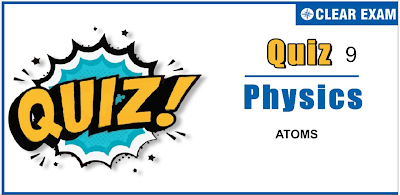## ATOMS QUIZ-9

JEE Advanced Physics Syllabus can be referred by the IIT aspirants to get a detailed list of all topics that are important in cracking the entrance examination. JEE Advanced syllabus for Physics has been designed in such a way that it offers very practical and application-based learning to further make it easier for students to understand every concept or topic by correlating it with day-to-day experiences. In comparison to the other two subjects, the syllabus of JEE Advanced for physics is developed in such a way so as to test the deep understanding and application of concepts.

Q1. A beam of 13.0 eV electrons is used to bombard gaseous hydrogen. The series obtained in emission spectra is/are
•  Lyman series
•  Balmer series
•  Bracket series
•  All of these
Solution
(d) As the electron beam is having energy of 13 eV, it can excite the atom to the states whose energy is less than or equal to 0.6 eV (13.6-13).E_5=0.544 eV and E_4=0.85 eV. So, the electron beam can excite the hydrogen gas maximum to 4th energy state, hence the transit electron can come back to ground state from either of three excited states, thus emitting Lyman, Balmer and Paschen series

Q2. The ratio of minimum to maximum wavelength in Balmer series is
•  5:9
•  5:36
•  1:4
•  3:4
Q3. An electron with kinetic energy E eV collides with a hydrogen atom in the ground state. The collision is observed to be elastic for
•   0<E<∞
•  0<E< 10.2 eV
•  0<E< 13.6 eV
•  0<E< 3.4 eV
Solution
(b) For an elastic collision to take place, there must be no loss in the energy of electron. The hydrogen atom will absorb energy from the colliding electron only if it can go from ground state to first excited state, i.e., from n=1 to n=2 state. For this, hydrogen atom must absorb energy E_2-E_1=-3.4-(-13.6)=10.2 eV So, if the electron possesses energy less than 10.2 eV, it would never lose it and hence collision would be elastic

Q4. The electric potential between a proton and an electron is given by V=V_0 In r/r_0 , where r_0 is a constant. Assuming Bohr’s model to be applicable, write variation of r_n with n,n being the principal quantum number?
•  r_n∝ n
•  r_n∝1/n
•  r_n∝ n^2
•  r_n∝1/n^2
Q5. Which of the following statement is true regarding Bohr’s model of hydrogen atom?
•  Orbiting speed of an electrons decreases as it falls to discrete orbits away from the nucleus
•  Radii of allowed orbits of electrons are proportional to the principal quantum number
•  Frequency with which electrons orbit around the nucleus in discrete orbits is inversely proportional to the cube of principal quantum number
•  Binding force with which the electron is bound to the nucleus increase as it shifts to outer orbits
Q6. Hydrogen atoms in a sample are excited to n=5 state and it is found that photons of all possible wavelengths are present, in the emission spactra. The minimum number of hydrogen atoms in the sample would be
•  5
•  6
•  10
•  INFINTE
Q7. The circumference of the second Bohr orbit of electron in hydrogen atom is 600 nm. The potential difference that must be applied between the plates so that the electrons have the de Broglie wavelength corresponding in this circumference is
•  10^(-5) V
•  5/3×10^(-5) V
•  5×10^(-5) V
•  3×10^(-5) V
Q8. The wavelength of the spectral line that corresponds to a transition in hydrogen atom from n=10 to ground state would be [In which part of electromagnetic spectrum this line lies?]
•  92.25 nm, ultraviolet
•  92.25 nm, infrared
•  86.95 nm, ultraviolet
•  97.65 nm, ultraviolet
Q9. A photon collides with a stationary hydrogen atom in ground state inelastically. Energy of the colliding photon is 10.2 eV. Almost instantaneously, another photon collides with same hydrogen atom inelastically with an energy of 15 eV. What will be observed by the detector?
•  Two photons of energy 10.2 eV
•  Two photon of energy 1.4 eV
•  One photon of energy 10.2 eV and one electron of energy 1.4 eV
•  One electron having kinetic energy nearly 11.6 eV
Solution
(d) Total energy received by the atom will be 25.2 eV. 13.6 eV energy is needed to remove the electron from the attraction of the nucleus. Rest of the energy will be almost available in the form of KE of electron

Q10. In hydrogen spectrum, the shortest wavelength in Balmer series is λ. The shortest wavelength in Brackett series will be
•  2λ
•  4λ
•  9λ
• 16λ#### Written by: AUTHORNAME

AUTHORDESCRIPTION## Want to know more

Please fill in the details below:

## Latest NEET Articles\$type=three\$c=3\$author=hide\$comment=hide\$rm=hide\$date=hide\$snippet=hide

Name

ltr
item
BEST NEET COACHING CENTER | BEST IIT JEE COACHING INSTITUTE | BEST NEET, IIT JEE COACHING INSTITUTE: Atoms - Quiz 9
Atoms - Quiz 9
https://1.bp.blogspot.com/-rD3rXGLx_C8/YONX6dLxLnI/AAAAAAAATdM/FCcToO6Xk3EsitRj8XiXwWPEEkP-IaNgwCLcBGAsYHQ/s400/9.png
https://1.bp.blogspot.com/-rD3rXGLx_C8/YONX6dLxLnI/AAAAAAAATdM/FCcToO6Xk3EsitRj8XiXwWPEEkP-IaNgwCLcBGAsYHQ/s72-c/9.png
BEST NEET COACHING CENTER | BEST IIT JEE COACHING INSTITUTE | BEST NEET, IIT JEE COACHING INSTITUTE
https://www.cleariitmedical.com/2021/07/atoms-quiz-9.html
https://www.cleariitmedical.com/
https://www.cleariitmedical.com/
https://www.cleariitmedical.com/2021/07/atoms-quiz-9.html
true
7783647550433378923
UTF-8